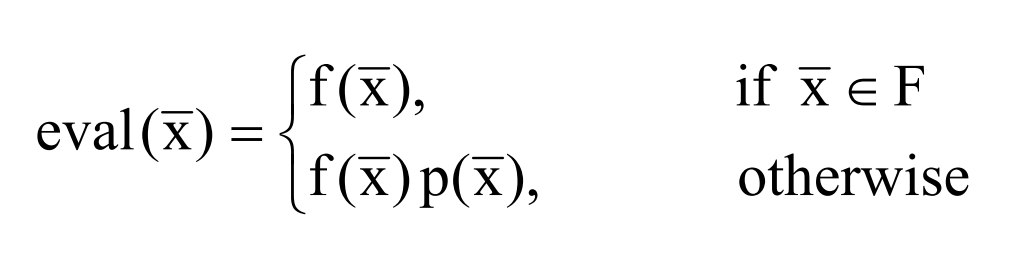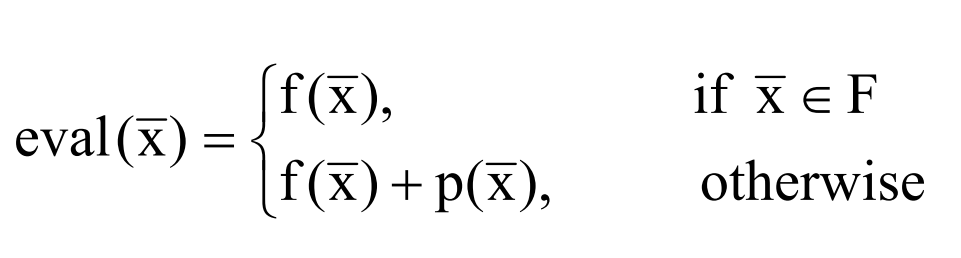## Introduction

The penalty method is a class of algorithms for solving constrained optimization problems. In a penalty method, a constrained optimization problem is replaced by a series of unconstrained problems whose solutions ideally converge to that of the original constrained problem.

Optimization algorithms for image compression can use penalty functions to choose how to compress zones of color to single representative values.

## Constrained Optimization

The following form of a constrained optimization problem is usually written as a nonlinear optimization problemThe vector of solutions t 1 n x = (x ,…, x ), the feasible region F, and the whole space S are given. In addition, there are m-q inequality constraints on solutions. F(x) is usually referred to as the objective function or criterion function. In the problem, linear constraint functions and objective functions can exist. The solution to the problem is a vector x that satisfies all constraints. A feasible region consists of all feasible solutions. Inequality constraints that satisfy g (x) 0 i = are said to be active at x. Nonlinear programming problem is to find a point x F * * such that f(x) f(x) * ≤ for all x ∈ F.

## Penalty Function

Penalty method transforms constrained problems into unconstrained ones in two ways. The first way is to use additive form:The penalty term (x) is represented by p. If there is no violation, p will be zero and (x) positive otherwise. Through this conversion, the overall objective function is now eval (x), which serves as an evaluation functionIf no violation occurs, p is one and bigger than one, otherwise (x).

Two types of penalty functions are commonly used in classical optimization: interior and exterior penalty functions## Death penalty

In this case, there will never be any unfeasible solutions in the population: P(x) = +∞, x ∈S − F . There will never be any unfeasible solutions in the population. This method is likely to work well if a feasible search space is convex or a reasonable portion of the whole search space. If the problem is highly constrained, however, the algorithm will spend a lot of time searching for too few solutions. Furthermore, considering only the points in the feasible region of the search space prevents finding better solutions.

## Static Penalty

The penalty parameters used by this group don’t depend on the current generation number, and a constant penalty is applied to infeasible solutions. Homaifar et al. proposed a static penalty approach in which users define levels of violation. The steps are:

Create l levels of violation for each constraint

Calculate the penalty coefficient Rij (i=1,…,l; j=1,…,m) for each level of violation and each constraint. In general, bigger coefficients are assigned to higher levels of violation.

Create a random population that includes both feasible and infeasible individuals,

Assessing individuals based on

This method has the disadvantage that a large number of parameters must be set. It is necessary to set m(2l+1) parameters in total for m constraints in this method. The total number of parameters should be set to 45 when m=5 constraints and l=4 levels of violation. Michaelewicz shows that the quality of solutions is highly dependent on these parameters.

## Dynamic Penalty

As the generation grows, the penalty increases. Changes in * and * values greatly affect the quality of a possible solution. The sensitivity of the method for different values of C is not explained. Michalewicz  gave some examples to show that these parameter values result in premature convergence even though Joines and Houck  stated that they selected C=0.5 and *=*=2. Furthermore, he showed that the method converges to an infeasible solution or a solution so far from being optimal.

## Annealing Penalties

In this group are some methods based on annealing algorithms. Michael Malewicz and Attia  developed a method (GENECOP II) based on simulated annealing. This method involves the following steps:

• Separate all constraints into four categories: linear equations, linear inequalities, nonlinear equations, and nonlinear inequalities,
• Find a random single point that satisfies linear constraints and use it as a starting point. Initially, the population is made up of copies of this point, Create an active constraint A consisting of nonlinear equations and violated nonlinear inequalities

## Implementation

Here we are using Iterative Solution from Nm dev Library to deal with the constrained optimization problem using a multivariate function.

				
%use s2
import dev.nm.solver.IterativeSolution

// construct a constrained optimization problem
fun problem(): ConstrainedOptimProblem {
// An example multivariate function.
val f: RealScalarFunction = object : AbstractUnivariateRealFunction() {
override fun evaluate(x: Double): Double {
return Math.pow(x+4.0, 2.0)
}
}


				
// A less than constraint for constrained optimization.
val c2: LessThanConstraints = LinearLessThanConstraints(
DenseMatrix(arrayOf(doubleArrayOf(-1.0))),
DenseVector(-10.0)
);


				
// construct an optimization problem
val problem = ConstrainedOptimProblemImpl1(f, null, c2)
return problem
}


				
// Solve a general constrained optimization using BFGS.
val gamma = 1e30
val optim = PenaltyMethodMinimizer(
PenaltyMethodMinimizer.DEFAULT_PENALTY_FUNCTION_FACTORY,
gamma,
BFGSMinimizer( // an optimizer to use in conjunction with PenaltyMethodMinimizer
false,
1e-8, // epsilon
200) // max number of iterations


				
val p = problem()
val minimizer: IterativeSolution = optim.solve(p)
val xmin: Vector? = minimizer.search(DenseVector(0.0)) // initial guess = (0.0)
val fxmin: Double = p.f().evaluate(xmin)
println("f($xmin) =$fxmin")



## Output:## Conclusion

Optimization problems in the real world have constraints that must be satisfied by the solution. A variety of constraint handling methods have been proposed by many researchers. Each method has its own advantages and disadvantages. The most popular constraint handling method is the penalty function method. In this article we discuss these methods and discuss their strengths and weaknesses. There is no one method that is the best for every problem. In most methods, the main challenge is setting the appropriate values for the penalty parameters. As a result, users have to experiment with different values of penalty parameters.